# NCERT Solutions for Class 7 Maths Chapter 7 Congruence of Triangles| PDF Download

On this page you will find Chapter 7 Congruence of Triangles Class 7 Maths NCERT Solutions which will help in understanding the important points inside the chapter properly. Here you can also download PDF of Chapter 7 Congruence of Triangles NCERT Solutions for Class 7 Maths that will increase concentration among students. It can be used to enrich knowledge and make lessons for learners more exciting.

Class 7 Maths NCERT Solutions will help in solving the difficulties that lie ahead with ease. These NCERT Solutions are prepared as per the accordance of latest CBSE guidelines so you can score maximum marks.Exercise 7.1

1. Complete the following statements:
(a) Two line segments are congruent if _______________.
(b) Among two congruent angles, one has a measure of 70o, the measure of other angle is __________.
(c) When we write ∠A = ∠B, we actually mean _______________.

(a) they have the same length
(b) 70°
(c) m∠A = m∠B

2. Give any two real time examples for congruent shapes.

(i) Two footballs
(ii) Two teacher’s tables

3. If ΔABC ≌  ΔFED under the correspondence ABC↔FED, write all the corresponding congruent parts of the triangles.Given: ΔABC ≌  ΔFED.
The corresponding congruent parts of die triangles are:
(i) ∠A ↔ ∠F
(ii) ∠B ↔ ∠E
(iii) ∠C ↔ ∠D4. If ΔDEF ≌  ΔBCA, write the part (s) of ΔBCA that correspond to: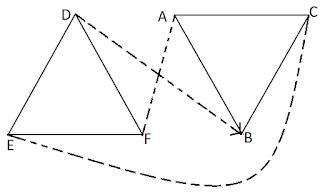Exercise 7.2

1. Which congruence criterion do you use in the following?
(a) Given: AC = DF, AB = DE, BC = EF
So ΔABC ≌ ΔDEF(b) Given: RP = ZX, RQ = ZY, ∠PRQ = ∠XZY
So ΔPQR ≌ ΔXYZ(c) Given: ∠ MLN = ∠ FGH, ∠ NML = ∠ HFG, ML = FG
So ΔLMN ≌ ΔGFH(d) Given: EB = BD, AE = CB, ∠A = ∠C = 90°
So AABE = ACDB(a) By SSS congruence criterion, since it is given that AC = DF, AB = DE, BC = EF
The three sides of one triangle are equal to the three corresponding sides of another triangle.
Therefore, ΔABC ≌ ΔDEF

(b) By SAS congruence criterion, since it is given that RP = ZX, RQ = ZY and ∠PRQ = ∠XZY
The two sides and one angle in one of the triangle are equal to the corresponding sides and the angle of other triangle.
Therefore, ΔPQR ≌ ΔXYZ

(c) By ASA congruence criterion, since it is given that ∠MLN = ∠FGH, ∠NML = ∠HFG, ML = FG.
The two angles and one side in one of the triangle are equal to the corresponding angles and side of other triangle.
Therefore, ΔLMN ≌ ΔGFH

(d) By RHS congruence criterion, since it is given that EB = BD, AE = CB, ∠A = ∠C = 90°
Hypotenuse and one side of a right angled triangle are respectively equal to the hypotenuse and one side of another right angled triangle.
Therefore, ΔABE ≌ ΔCDB.

2. You want to show that ΔART ≌ ΔPEN:
(a) If you have to use SSS criterion, then you need to show:
(i) AR =
(ii) RT =
(iii) AT =

(b) If it is given that ∠T = ∠N and you are to use SAS criterion, you need to have:
(i) RT = and
(ii) PN =

(c) If it is given that AT = PN and you are to use ASA criterion, you need to have:
(i) ?
(ii) ?(a) Using SSS criterion, ΔART ≌ ΔPEN
(i) AR = PE
(ii) RT= EN
(iii) AT = PN

(b) Given: ∠T = ∠N
Using SAS criterion, ΔART ≌ ΔPEN
(i) RT = EN
(ii) PN = AT

(c) Given: AT = PN
Using ASA criterion, ΔART ≌ ΔPEN
(i) ∠RAT = ∠EPN
(ii) ∠RTA = ∠ENP

3. You have to show that ΔAMP = ΔAMQ. In the following proof supply the missing reasons:Steps Reasons (i) PM = QM (i) (ii) ∠ PMA = ∠ QMA (ii) (iii) AM = AM (iii) (iv) ΔAMP ≌ ΔAMQ (iv)

 Steps Reasons (i) PM = QM (i) Given (ii) ∠ PMA = ∠ QMA (ii) Given (iii) AM = AM (iii) Common (iv) ΔAMP ≌ ΔAMQ (iv) SAS congruence rule

4. In ΔABC, ∠A = 30° ∠B = 40° and ∠C = 110°
In ΔPQR, ∠P = 30° ∠Q = 40° and ∠R = 110°.
A student says that ΔABC ≌ ΔPQR by AAA congruence criterion. Is he justified? Why or why not?

No, because the two triangles with equal corresponding angles need not be congruent. In such a correspondence, one of them can be an enlarged copy of the other.

5. In the figure, the two triangles are congruent. The corresponding parts are marked. We can write Δ RAT ≌ ?In the figure, given two triangles are congruent. So, the corresponding parts are:
A↔O
R↔W
T↔N
We can write, ΔRAT ≌ ΔWON    [By SAS congruence rule]

6. Complete the congruence statement: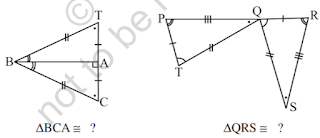In A BAT and ABAC, given triangles are congruent so the corresponding parts are:
B↔B
A↔A
T↔C
Thus, ΔBCA ≌ ΔBTA    |By SSS congruence rule]
In ΔQRS and ΔTPQ, given triangles are congruent so the corresponding parts are:
P↔R
T↔Q
Q↔S
Thus, ΔQRS ≌  ΔTPQ    [By SSS congruence rule]

7. In a squared sheet, draw two triangles of equal area such that:
(i) the triangles are congruent.
(ii) the triangles are not congruent.
What can you say about their perimeters?

In a squared sheet, draw ΔABC and ΔPQR. When two triangles have equal areas and
(i)In the above figure, ΔABC and ΔDEF have equal areas.
And also, ΔABC≌ΔDEF
So, we can say that perimeters of ΔABC and ΔDEF are equal.

(ii)In the above figure, ΔLMN and ΔOPQ
ΔLMN is not congruent to ΔOPQ
So, we can also say that their perimeters are not same.

8. Draw a rough sketch of two triangles such that they have five pairs of congruent parts but still the triangles are not congruent.

Let us draw two triangles PQR and ABC.All angles are equal, two sides are equal except one side. Hence, ΔPQR are not congruent to ΔABC.

9. If ΔABC and ΔPQR are to be congruent, name one additional pair of corresponding parts. What criterion did you use?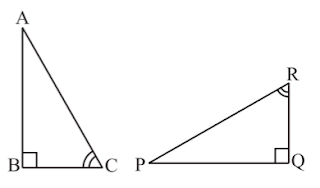A ABC and A PQR are congruent Then one additional pair is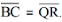Given: ∠B = ∠Q = 90°
∠C/BC = ∠R/QR
Therefore, ΔABC ≌ ΔPQR    [By ASA congruence rule]

10. Explain, why ΔABC ≌ ΔFED.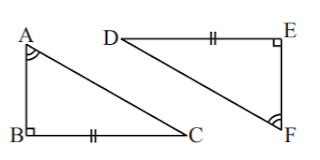Given: ∠A = ∠F, BC = ED, ∠B = ∠E
In ΔABC and ΔFED,
∠B = ∠E = 90°
∠A = ∠F
BC = ED
Therefore, ΔABC ≌ ΔFED    [By RHS congruence rule]

## NCERT Solutions for Class 7 Maths Chapter 7 Congruence of Triangles

Chapter 7 Congruence of Triangles NCERT Solutions will help you get a deeper understanding of various topics. If two objects are of exactly the same shape and size, they are said to be congruent. The relation between two congruent objects is called congruence.

• If two line segments have the same or equal length, they are congruent. Also, if two line segments are congruent, then they have the same length.

• If two angles have the same measure, they are congruent. Also, if two angles are congruent, their measures are the same.

Below you will also find exercisewise Class 7 NCERT Solutions which help you in finding specific question that you're looking for. These NCERT Solutions can serve as beneficial tool that can be used to recall various questions any time.

Through the help of NCERT Solutions for Class 7, you can figure out the latest marking scheme and prepare your answers as per the demand/devise your own strategy. It is very challenging to score good marks in tests that is why we have prepared NCERT Solutions.

### NCERT Solutions for Class 7 Maths Chapters:

 Chapter 1 Integers Chapter 2 Fractions and Decimals Chapter 3 Data Handling Chapter 4 Simple Equations Chapter 5 Lines and Angles Chapter 6 The Triangle and its Properties Chapter 8 Comparing Quantities Chapter 9 Rational Numbers Chapter 10 Practical Geometry Chapter 11 Perimeter and Area Chapter 12 Algebraic Expressions Chapter 13 Exponents and Powers Chapter 14 Symmetry Chapter 15 Visualising Solid Shapes

FAQ on Chapter 7 Congruence of Triangles

#### How many exercises are there in Chapter 7 Congruence of Triangles Class 7 Maths NCERT Solutions?

There are only two exercises in the Chapter 7 Congruence of Triangles which is going to help in building basics and getting a deeper understanding of various topics. These Class 7 NCERT Solutions will help you in improving the marks in the examinations and have edge over your classmates.

#### What do you mean by SAS congruence criterion?

Two triangles are congruent if two sides and the included angle of one triangle are equal to the corresponding two sides and the included angle of the other triangle.

#### What do you mean by RHS congruence criterion?

Two right-angled triangles are congruent if the hypotenuse and a side of one triangle are equal to the hypotenuse and the corresponding side of the other triangle.

#### What do you mean by ASA congruence criterion?

Two triangles are congruent if two angles and the included side of one triangle are equal to the corresponding two angles and the included side of the other triangle.